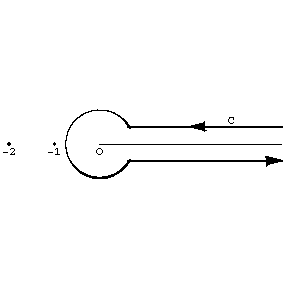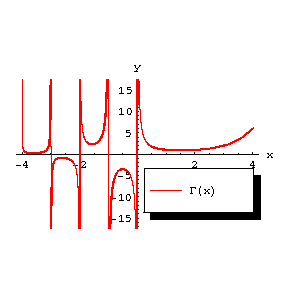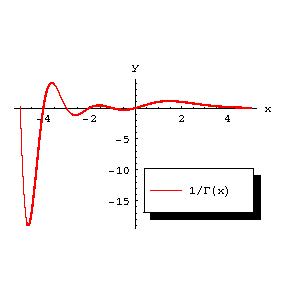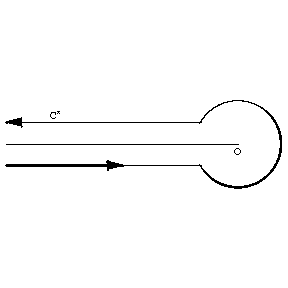# Difference between revisions of "Talk:Gamma-function"

$\Gamma$-function

$\newcommand{\Re}{\mathop{\mathrm{Re}}}$

A transcendental function $\Gamma(z)$ that extends the values of the factorial $z!$ to any complex number $z$. It was introduced in 1729 by L. Euler in a letter to Ch. Goldbach, using the infinite product $$\Gamma(z) = \lim_{n\rightarrow\infty}\frac{n!n^z}{z(z+1)\ldots(z+n)} = \lim_{n\rightarrow\infty}\frac{n^z}{z(1+z/2)\ldots(1+z/n)},$$ which was used by L. Euler to obtain the integral representation (Euler integral of the second kind, cf. Euler integrals) $$\Gamma(z) = \int_0^\infty x^{z-1}e^{-x} \rd x,$$ which is valid for $\Re z > 0$. The multi-valuedness of the function $x^{z-1}$ is eliminated by the formula $x^{z-1}=e^{(z-1)\ln x}$ with a real $\ln x$. The symbol $\Gamma(z)$ and the name gamma-function were proposed in 1814 by A.M. Legendre.

If $\Re z < 0$ and $-k-1 < \Re z < -k$, $k=0,1,\ldots$, the gamma-function may be represented by the Cauchy–Saalschütz integral: $$\Gamma(z) = \int_0^\infty x^{z-1} \left( e^{-x} - \sum_{m=0}^k (-1)^m \frac{x^m}{m!} \right) \rd x.$$ In the entire plane punctured at the points $z=0,-1,\ldots$, the gamma-function satisfies a Hankel integral representation: $$\Gamma(z) = \frac{1}{e^{2\pi iz} - 1} \int_C s^{z-1}e^{-s} \rd s,$$ where $s^{z-1} = e^{(z-1)\ln s}$ and $\ln s$ is the branch of the logarithm for which $0 < \arg\ln s < 2\pi$; the contour $C$ is represented in Fig. a. [FIXME] It is seen from the Hankel representation that $\Gamma(z)$ is a meromorphic function. At the points $z_n = -n$, $n=0,1,\ldots$ it has simple poles with residues $(-1)^n/n!$.Figure: g043310a

## Fundamental relations and properties of the gamma-function.

1) Euler's functional equation: $$z\Gamma(z) = \Gamma(z+1),$$ or $$\Gamma(z) = \frac{1}{z\ldots(z+n)}\Gamma(z+n+1);$$ $\Gamma(1)=1$, $\Gamma(n+1) = n!$ if $n$ is an integer; it is assumed that $0! = \Gamma(1) = 1$.

2) Euler's completion formula: $$\Gamma(z)\Gamma(1-z) = \frac{\pi}{\sin \pi z}.$$ In particular, $\Gamm(1/2)=\sqrt{\pi}$; $$\Gamma(n+1/2) = \frac{1.3\ldots(2n-1)}{2^n}\sqrt{\pi}$$ if $n>0$ is an integer;

 

3) Gauss' multiplication formula:

 

If , this is the Legendre duplication formula.

4) If  or , then  can be asymptotically expanded into the Stirling series:

 
 

where  are the Bernoulli numbers. It implies the equality

 
 

In particular,

 

More accurate is Sonin's formula :

 

5) In the real domain,  for  and it assumes the sign  on the segments ,  (Fig. b).Figure: g043310b

The graph of the function .

For all real  the inequality

 

is valid, i.e. all branches of both  and  are convex functions. The property of logarithmic convexity defines the gamma-function among all solutions of the functional equation

 

up to a constant factor.

For positive values of  the gamma-function has a unique minimum at  equal to . The local minima of the function  form a sequence tending to zero as .Figure: g043310c

The graph of the function .

6) In the complex domain, if , the gamma-function rapidly decreases as ,

 

7) The function  (Fig. c) is an entire function of order one and of maximal type; asymptotically, as ,

 

where

 

It can be represented by the infinite Weierstrass product:

 

which converges absolutely and uniformly on any compact set in the complex plane ( is the Euler constant). A Hankel integral representation is valid:

 

where the contour  is shown in Fig. d.Figure: g043310d



G.F. Voronoi  obtained integral representations for powers of the gamma-function.

In applications, the so-called poly gamma-functions — -th derivatives of  — are of importance. The function (Gauss' -function)

 
 

is meromorphic, has simple poles at the points  and satisfies the functional equation

 

The representation of  for  yields the formula

 

where

 

This formula may be used to compute  in a neighbourhood of the point .

For other poly gamma-functions see . Theincomplete gamma-function is defined by the equation

 

The functions  and  are transcendental functions which do not satisfy any linear differential equation with rational coefficients (Hölder's theorem).

The exceptional importance of the gamma-function in mathematical analysis is due to the fact that it can be used to express a large number of definite integrals, infinite products and sums of series (see, for example, Beta-function). In addition, it is widely used in the theory of special functions (thehypergeometric function, of which the gamma-function is a limit case, cylinder functions, etc.), in analytic number theory, etc.